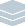Wolfram Archive

# Mathematical Tables(Abramowitz & Stegun, Gradshteyn & Ryzhik, DLMF, ...)
For more than 400 years, mathematical tables were a mainstay of applied mathematics, and indeed their replacement was an early motivation for the whole development of computers. One of the achievements of Mathematica is finally to render traditional mathematical tables obsolete. For within Mathematica are the algorithms to compute all standard tabulated mathematical functions, as well as integrals, sums, etc.—not just at the particular values that would be found in tables, but for any values of any parameters, and with any degree of precision. And when results are produced with Mathematica, they can immediately be used for further computation or visualization—for the first time allowing higher mathematical functions to become part of standard computational practice.
For over two decades, Wolfram Research has been the world leader in R&D on mathematical functions and symbolic calculus. Building on Mathematica's ever-increasing web of numeric and symbolic capabilities—as well as on unique automated algorithm discovery methods—Wolfram Research has developed new algorithms at an unprecedented rate and made them available in Mathematica. In addition, using Mathematica, Wolfram Research has produced the world's largest collection of results about mathematical functions: The Wolfram Functions Site.
Mathematica Features Covering Mathematical Tables:
Key Advantages of Mathematica Compared to Mathematical Tables:
Interoperability with Mathematical Tables:
Interesting Tidbits:
• Most mathematical tables are included in the automated quality tests for Mathematica
• Mathematica has found many errors in printed tables
• Many of the leading authors of mathematical tables have contributed to Mathematica
• Wolfram Mathematica Online Integrator has been running since 1996 and has computed nearly a billion integrals
• Mathematica is used as the test for FPUs and new mathematical systems libraries
• A 1996 NIST study already confirmed Mathematica to have by far the broadest mathematical function coverage